# 20. 深度学习 - 多层神经网络Hi，你好。我是茶桁。

\begin{align*} Sigmoid: f(x) = \sigma (x) = \frac{1}{1+e^{-x}} \end{align*}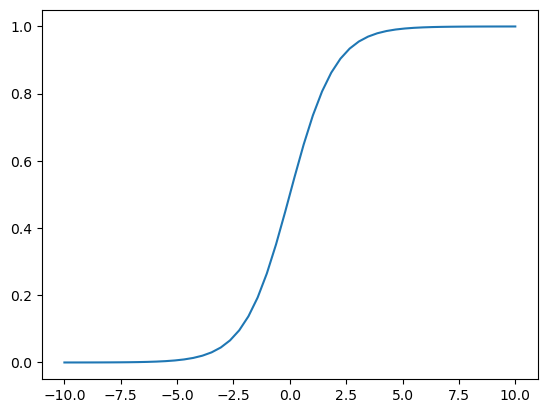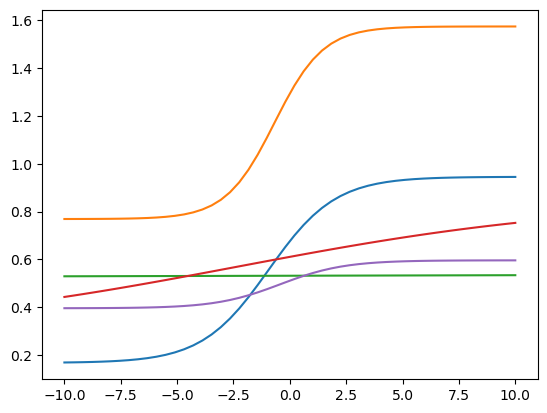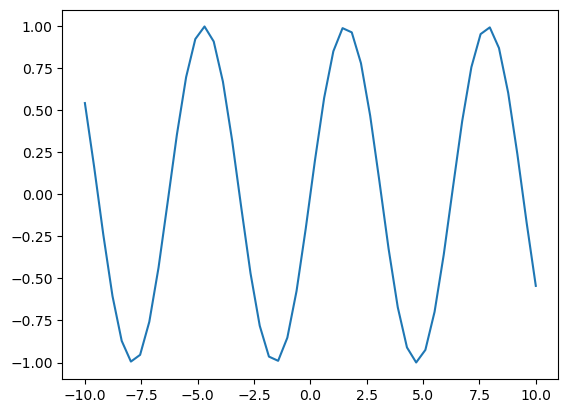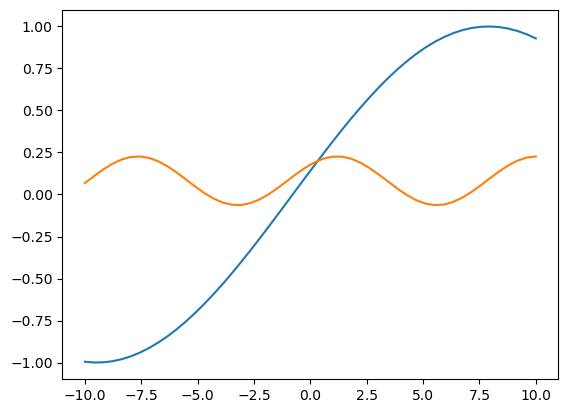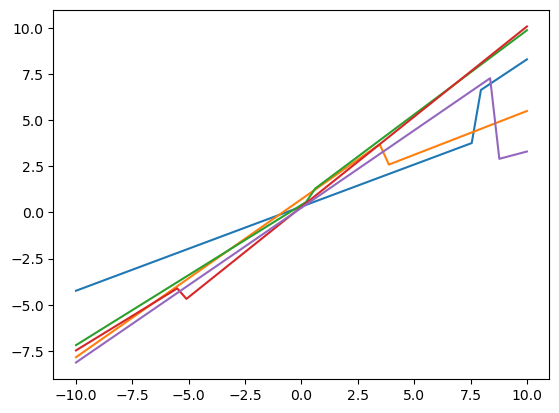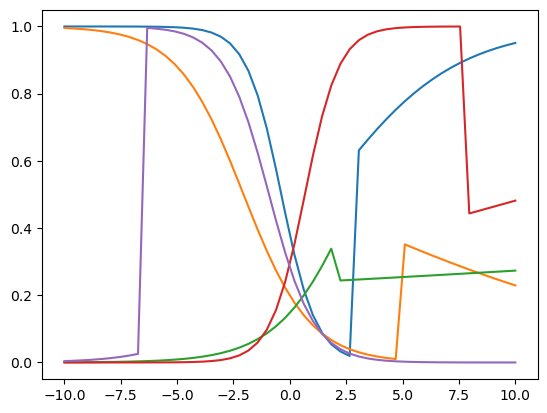\begin{align*} loss & = \frac{1}{N}(y_i - \hat y)^2 \\ & = \frac{1}{N} \begin{bmatrix} y_1 - (k_2 \frac{1}{1+e^{-(k_1x+b_1)}}+b_2) \end{bmatrix} ^2 \end{align*}

$\frac{1}{N}[l_2(\sigma(l_1(x))) -y_1]^2$

$\frac{\partial loss}{\partial l_2} \cdot \frac{\partial l_2}{\partial \sigma} \cdot \frac{\partial \sigma}{\partial l_1} \cdot \frac{\partial l_1}{\partial k_1}$

$\frac{\partial loss}{\partial l_2} \cdot \frac{\partial l_2}{\partial \sigma} \cdot \frac{\partial \sigma}{\partial l_1} \cdot \frac{\partial l_1}{\partial b_1}$

$\frac{\partial loss}{\partial l_2}$其实就等于$\frac{2}{N}(l_2 - y_1)$

\begin{align*} \frac{2}{N}(l_2 - y_1) \cdot k_2 \cdot \sigma \cdot (1 - \sigma) \cdot x \end{align*}

\begin{align*} & \{k_1, b_1, x\} \to l_1 \to \sigma, \\ & \{\sigma, k_2, b_2\} \to l_2, \\ & \{l_2, y_{true}\} \to loss \\ & \to 表示的是'输出到'的关系。 \end{align*}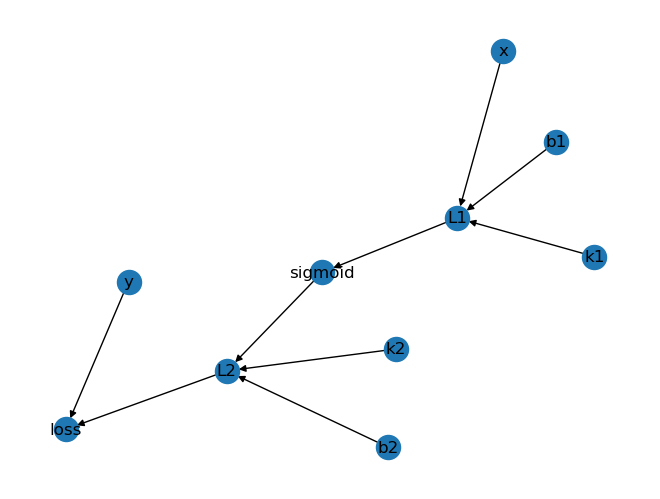20. 深度学习 - 多层神经网络

https://hivan.me/20. 深度学习 - 多层神经网络/

Hivan Du

2023-11-13

2023-11-25